# MCQ Questions for Class 10 Maths Chapter 2 Polynomials with Answers

Students can access the NCERT MCQ Questions for Class 10 Maths Chapter 2 Polynomials with Answers Pdf free download aids in your exam preparation and you can get a good hold of the chapter. Use MCQ Questions for Class 10 Maths with Answers during preparation and score maximum marks in the exam. Students can download the Polynomials Class 10 MCQs Questions with Answers from here and test their problem-solving skills. Clear all the fundamentals and prepare thoroughly for the exam taking help from Class 10 Maths Chapter 2 Polynomials Objective Questions.

## Polynomials Class 10 MCQs Questions with Answers

Students are advised to solve the Polynomials Multiple Choice Questions of Class 10 Maths to know different concepts. Practicing the MCQ Questions on Polynomials Class 10 with answers will boost your confidence thereby helping you score well in the exam.

Explore numerous MCQ Questions of Polynomials Class 10 with answers provided with detailed solutions by looking below.

Question 1.
If a polynomial p(y) is divided by y + 2, then which of the following can be the remainder:
(a)y + 1
(b)2y + 3
(c) 5
(d)y – 1

When p(y) is divided by y + 2, then the degree of remainder < deg of (y + 2)

Question 2.
If a polynomial p(x) is divided by b – ax; the remainder is the value of p(x) at x =
(a) a
(b) $$\frac{b}{a}$$
(c) $$\frac{- b}{a}$$
(d) $$\frac{a}{b}$$

Answer: (b) $$\frac{b}{a}$$
b – ax = 0
x = $$\frac{b}{a}$$

Question 3.
If the polynomials ax³ + 4x² + 3x – 4 and x³ – 4x + a, leave the same remainder when divided by (x – 3), then value of a is :
(a) 2b
(b) – 1
(c) 1
(d) – 2b

p(x) = ax³ + 4x² + 3x – 4
q(x) = x³ – 4x + a
p(3) = q(3)
a = – 1

Question 4.
If p(x) = 2x⁴ – ax³ + 4x² + 2x + 1 is a. multiple of 1 – 2x, then find the value of a :
(a) 25
(b) $$\frac{1}{2}$$
(c)$$\frac{- 1}{2}$$
(d) 8

p(x) is a multiple of 1 – 2x.
1 – 2x is a factor of p(x)

Question 5.
If -2 is a zero of p(x) = (ax³ + bx² + x – 6) and p(x) leaves a remainder 4 when divided by (x – 2), then the values of a and b are (respectively):
(a)a = 2,b = 2
(b) a = 0,b = – 2
(c) a = 0, b = 2
(d) a = 0, b = 0

Answer: (c) a = 0, b = 2
If – 2 is a zero =>
p(- 2) = 0
=> – 2a + b = 2
Also, p(2) = 4
2a + b = 2=>a = 0and b = 2

Question 6.
If x101 + 1001 is divided by x + 1, then remainder is:
(a) 0
(b) 1
(c) 1490
(d) 1000

p(x) is divided by x + 1
p(- 1) = (-1101) + 1001 = 1000

Question 7.
If one zero of a polynomial p(x) = ax² + bx + c(a ≠ 0) is zero, then, which of the following is correct:
(a) b = 0
(b) c = 0
(c) other zero is also zero
(d) Nothing can be said about p(x).

let ,α = 0
Product of the roots = αs = 0
= $$\frac{c}{a}$$ = 0

Question 8.
If α, s are the zeroes of x² – lx + m, then
$$\frac{α}{s}$$ + $$\frac{s}{α}$$
(a) $$\frac{l² – 2m}{m}$$
(b) $$\frac{l² + 2m}{m}$$
(c) $$\frac{l – 2m}{m}$$
(d) l² – 2m

Answer: (a) $$\frac{l² – 2m}{m}$$
α + s = l
αs = m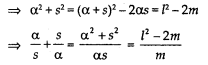Question 9.
sum of the squares of the zeroes of the polynomial p(x) = x² + 7x – k is 25, find k.
(a) 12
(b) 49
(c) – 24
(d) – 12

p(x) = x² + 7x – k
let α,s be the zeroes
α + s = – 7
αs = – k
α² + s² = 25
(α² + s) – 2αs = 25
49 + 2k = 25
k = -12

Question 10.
If one zero of 3x² – 8x + 2k + 1 is seven times the other, find k.
(a) $$\frac{2}{3}$$
(b) $$\frac{1}{3}$$
(c) $$\frac{4}{3}$$
(d) $$\frac{5}{3}$$

Answer: (a) $$\frac{2}{3}$$
α + 7α = 8α = $$\frac{8}{3}$$
α = $$\frac{1}{3}$$
k = $$\frac{2}{3}$$

Question 11.
Let, α, s, v be the zeroes of x³ + 4x² + x- 6 such that product of two of the zeroes is 6. Find the third zero.
(a) 6
(b) 2
(c) 4
(d) 1

α s v = 6,
αs = 61
=> v = 6

Question 12.
If a, s are the zeroes of x² – 8x + λ, such
that α – s = 2, then X =
(a) 8
(b) 22
(c) 60
(d) 15

α + s = 8,
αs = λ
α – s = 2
=> (α – s)2 = 4
=> α²+s²-2αs = 4
=> (α + s)² – 4as = 4
=> 64 — 4λ = 4
=> 4λ. = 60
=> X = 15

Our synthetic division calculator shows you step-by-step how to divide polynomials with help of the synthetic division method.

Question 13.
Find a and b so that the polynomial 6x⁴ + 8x³ – 5x² + ax + b is exactly divisible by 2x² – 5.
(a) a = 20, b = – 25
(b) a = 4, b = – 5
(c) a = 20, b = 5
(d) a = – 20, b = – 25

Answer: (d) a = – 20, b = – 25
Divide the given polynomial by 2×2 – 5 get the remainder as (20 + a)x + (b + 25) which should be zero

Question 14.
If α, s are the zeroes of p(x) = 2x² – 5x + 7, write a polynomial with zeroes 2α+3s and 3α+2s.
(a) k(x² + $$\frac{5}{2}$$x – 41)
(b) k(x² – $$\frac{5}{2}$$x + 41)
(c) k(x² – $$\frac{5}{2}$$x – 41)
(d) k(- x² + $$\frac{5}{2}$$x + 41)

Answer: (b) k(x² – $$\frac{5}{2}$$x + 41)
α + s = $$\frac{5}{2}$$
αs = $$\frac{7}{2}$$
k(x² – $$\frac{5}{2}$$x + 41)

Question 15.
If sum of the two zeroes of a cubic polynomial x³ – ax² + bx – c, is zero, then which of the following is true:
(a) ab = c
(b) a – b = c
(c) ab = $$\frac{c}{2}$$
(d) a = $$\frac{b}{c}$$

Let, α, s, v be the roots =α + s + v = a
v = a
now v is a zero
ab = c

Question 16.
If a, s are the zeroes of p(x) = 2x² + 5x + k such that, α²+ s²+ αs = $$\frac{21}{4}$$, then k equals,
(a) 12
(b) 4
(c) 2
(d) – 12

α + s = – $$\frac{5}{2}$$
αs = $$\frac{k}{2}$$
α² + s² +αs = $$\frac{21}{4}$$
(α + s)² – αs = $$\frac{21}{4}$$
$$\frac{25}{4}$$ – $$\frac{k}{2}$$ = $$\frac{21}{4}$$
k = 2

Question 17.
If α, s are the zeroes of x² + px + q, then a polynomial having zeroes $$\frac{1}{α}$$ and $$\frac{1}{s}$$ is,
(a) x² + px + q
(b) x² + qx + p
(c) px² + qx + 1
(d) qx² + px +1

Answer: (d) qx² + px +1
α + s = – p
αs = q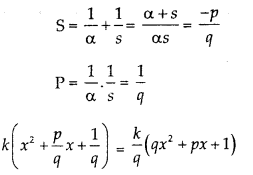Question 18.
Find the number of zeros in the graph given:(a) 3
(b) 2
(c) 1
(d) 0

Since the graph meets X-axis at two points -2 and 1, thus it has 2 zeroes.

Question 19.
Write the zero of the polynomial p(x), whose graph is given :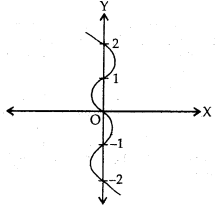(a) 1
(b) 0
(c) – 1
(d) – 2

Since the graph meets X-axis at x = 0
=> Zero of p(x) is ‘O’ => Correct option is (b).

Question 20.
If α, s, v are the zeros of the polynomial 2x³ – x² + 3x -1, find the value of (αsv) + (αs + sv + vα).
(a) 2
(b) $$\frac{3}{2}$$
(c) $$\frac{1}{2}$$
(d) 0

p(x) = 2x³ – x² + 3x -1
αsv = – d/a = $$\frac{1}{2}$$
αs + sv + vα = c/a = $$\frac{3}{2}$$
αs + sv + vα + αsv = $$\frac{3}{2}$$ + $$\frac{1}{2}$$ = 2

Question 21.
If the zeros of the polynomial x³ – 3x² + x +1 are p – q,p and p + q. Find the value of q.
(a) 1
(b) 0
(c) 2
(d) ±√2

x³ – 3x² + x +1
zeroes are p – q,p,p + q
sum of zeroes = (p – q) + p + (p + q)
= 3p
= 3
α + s + v = $$\frac{- b}{a}$$
further = αs + sv + vα = $$\frac{c}{a}$$
(p – q) p + p(p + q) + (p – q)(p + q)=1
q = ±√2

Question 22.
(a) at least 2 zeros
(b) exactly 2 zeros
(c) at most 2 zeros
(d) exactly 1 zero

Answer: (c) at most 2 zeros
A quadratic polynomial has atmost two zeroes.

Question 23.
Which of the following Linear Graphs has no zero?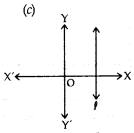Answer:as it does not meet X axis.

Question 24.
If α, s are the roots of cx² – bx + a = 0 (c 0), then α + s is:
(a) $$\frac{- b}{a}$$
(b) $$\frac{b}{a}$$
(c) $$\frac{c}{a}$$
(d) $$\frac{b}{c}$$

Answer: (d) $$\frac{b}{c}$$
sum of the roots = – $$\frac{coefficient of x}{coefficient of x²}$$ = $$\frac{b}{c}$$

Question 25.
If P(x) and D(r) are any two polynomials such that D(x) ≠ 0, there exists unique polynomial Q(x) and R(x) such that, P(x) = D(x). Q(x) + R(x) where :
(a) R(x) = 0 and deg R(x) > deg Q(x)
(b) R(x) = 0 or deg R(x) > deg D(x)
(c) deg R(x) < deg Q(x)
(d) R(x) = 0 or deg R(x) < deg D(x)

Answer: (b) R(x) = 0 or deg R(x) > deg D(x)
division algorithm

Question 26.
When we divide x³ + 5x + 7 by x⁴ – 7x² – 6 then quotient and remainder are (respectively):
(a) 0,x³ + 5x + 7
(b) x, 2x + 3
(c) 1,x⁴ – 7x²-6
(d) x², 4x – 9

Answer: (a) 0,x³ + 5x + 7
Degree of the divisor is more than the degree of the dividend = quotient is zero and the remainder is x³ + 5x + 7

Question 27.
The value of b, for which 2x³ + 9x² – x – b is exactly divisible by 2x + 3 is:
(a) -15
(b) 15
(c) 9
(d) – 9

when 2x³ + 9x² – x – b is divided by 2x + 3, remainder is – b + 15

Question 28.
If α and s are two zeros of the polynomial p(x), then which of the following is a factor of p(x):
(a) (x – α)(x – s)
(b) (x + α) (x + s)
(c) k(x – α)
(d) k(x- s)

Answer: (a) (x – α)(x – s)
if α, s are the zeros of p(x), then (x – α)(x – s) is a factor of p(x).

Question 29.
Find a cubic polynomial with the sum, sum of the product of its zeros taken two at a time and the product of its zeros as -2, +5, -3, respectively.
(a) 2x³ + 5x² + x + 3
(b) 4x³ + 5x² – 3x + 7
(c) x³ + 2x² + 5x + 3
(d) 2x³ + 5x² + 3x + 1

Answer: (c) x³ + 2x² + 5x + 3
Let the polynomial be ax³ + bx² + cx + d
– b/a = – 2
c/a = 5
– d/a = – 3
a = 1, b = 2, c = 5 and d = 3
required polynomial is x³ + 2x² + 5x + 3

Question 30.
Write a polynomial with zeros 1, – 1 and 1.
(a) x³ + x² + x + 1
(b) x³ – x² + x + 1
(c) x³ – x² – x – 1
(d) x³ – x² – x + 1

Answer: (d) x³ – x² – x + 1
zeros are 1, – 1 and 1.
required polynomial is
k(x – 1)(x +1)(x – 1)
= x³ – x² – x + 1

Question 31.
The graph of a polynomial is as shown, find the polynomial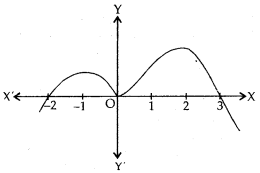(a) k(x² – x – 6)
(b) k(x³ + x² + 6x)
(c) k(x³ – x² – 6x)
(d) k(x³ – 6x)

Answer: (c) k(x³ – x² – 6x)
zeros are – 2,0, and 3
required polynomial = k(x – 2)(x – 0)(x – 3)
= k(x³ – x² – 6x)

Question 32.
If α, s and v are the zeroes of the polynomial 2x³ – x² + 3x – 1, find the value of => (as + sv + va + asv )²
(a) $$\frac{3}{2}$$
(b) $$\frac{5}{2}$$
(c) $$\frac{1}{2}$$
(d) 4

αs + sv + vα + αsv = $$\frac{3}{2}$$ + $$\frac{1}{2}$$ = 2
(αs + sv + vα + αsv )² = 4

Question 33.
If 2 ± √3 are the two zeros of a polynomial then the following is a factor:
(a) x² – 4x + 1
(b) x² + 4x – 1
(c) 4x² + x – 1
(d) 4x² – x + 1

Answer: (a) x² – 4x + 1
If a, s are the zeroes => (x – α) (x – s) is a factor
=> (x – (2 + √3)) (x – (2 – √3))is a factor
=> x2 – 4x + 1 is a factor.

Question 34.
If 2 is a zero of p(x) = x² + 3x + k, find k:
(a) 10
(b) 5
(c) – 3
(d) – 10

p(x) = x² + 3x + k
p(2) = 0
=>4 + 6 + k = 0
=k = – 10

Question 35.
Given that two of the zeroes of the
polynomial, x³ + px² + rx + s are 0, then third zero
(a) 0
(b) $$\frac{p}{r}$$
(c) $$\frac{- p}{r}$$
(d) $$\frac{p}{q}$$

Answer: (c) $$\frac{- p}{r}$$
Two zeroes are zero, let third zero = α
=> Sum of the roots = α + 0 + 0
$$\frac{Coefficient of x²}{Coefficient of x³}$$

Question 36.
Given that one of the zeroes of the
polynomial ax³ + bx² + cx + d is zero, then the product of the other two zeroes is:
(a) $$\frac{- c}{a}$$
(b) $$\frac{c}{a}$$
(c) 0
(d) $$\frac{- b}{a}$$

Answer: (b) $$\frac{c}{a}$$
αs + sv + vα = $$\frac{c}{a}$$
now α = 0
0 + sv + 0 = $$\frac{c}{a}$$
sv = $$\frac{c}{a}$$

Question 37.
The number of polynomials having zeroes – 1 and – 5 is :
(a) 2
(b) 3
(c) 1
(d) More than 3.

n – number of polynomials can have zeroes -1 and -5.

Question 38.
The graph of the polynomial f(x) = 2x – 5 intersects the x – axis at
(a) ($$\frac { 5 }{ 2 }$$, 0)
(b) ($$\frac { -5 }{ 2 }$$, 0)
(c) ($$\frac { -5 }{ 2 }$$, $$\frac { 5 }{ 2 }$$)
(d) ($$\frac { 5 }{ 2 }$$, $$\frac { -5 }{ 2 }$$)

Answer: (a) ($$\frac { 5 }{ 2 }$$, 0)

Question 39.
If the zeroes of the quadratic polynomial Ax2 + Bx + C, C # 0 are equal, then
(a) A and B have the same sign
(b) A and C have the same sign
(c) B and C have the same sign
(d) A and C have opposite signs

Answer: (b) A and C have the same sign

Question 40.
The number of polynomials having zeroes as 4 and 7 is
(a) 2
(b) 3
(c) 4
(d) more than 4

Question 41.
If one of the zeroes of the cubic polynomial x3 + ax2 + bx + c is -1, then the product of the
other two zeroes is
(a) b – a + 1
(b) b – a – 1
(c) a – b + 1
(d) a – b – 1

Answer: (a) b – a + 1

Question 42.
The number of zeros of a cubic polynomial is
(a) 3
(b) at least 3
(c) 2
(d) at most 3

Question 43.
Find the quadratic polynomial whose zeros are 2 and -6
(a) x2 + 4x + 12
(b) x2 – 4x – 12
(c) x2 + 4x – 12
(d) x2 – 4x + 12

Answer: (c) x2 + 4x – 12

Question 44.
If 5 is a zero of the quadratic polynomial, x2 – kx – 15 then the value of k is
(a) 2
(b) -2
(c) 4
(d) – 4

Question 45.
The number of polynomials having zeroes as -2 and 5 is
(a) 1
(b) 2
(c) 3
(d) more than 3

Question 46.
The zeroes of the quadratic polynomial x2 + 1750x + 175000 are
(a) both negative
(b) one positive and one negative
(c) both positive
(d) both equal

Question 47.
If the zeroes of the quadratic polynomial x2 + (a + 1) x + b are 2 and -3, then
(a) a = -7, b = -1
(b) a = 5, b = -1
(c) a = 2, b = -6
(d) a – 0, b = -6

Answer: (d) a – 0, b = -6

Question 48.
Sum and the product of zeroes of the polynomial x2 +7x +10 is
(a) $$\frac { 10 }{ 7 }$$ and $$\frac { -10 }{ 7 }$$
(b) $$\frac { 7 }{ 10 }$$ and $$\frac { -7 }{ 10 }$$
(c) -7 and 10
(d) 7 and -10

Question 49.
If x = 2 and x = 3 are zeros of the quadratic polynomial x2 + ax + b, the values of a and b respectively are :
(a) 5, 6
(b) – 5, – 6
(c) – 5, 6
(d) 5, – 6

Question 50.
The zeroes of the quadratic polynomial 3x2 – 48 are
(a) both negative
(b) one positive and one negative
(c) both positive
(d) both equal

Answer: (b) one positive and one negative

Question 14.
The zeroes of the quadratic polynomial x2 + kx + k, k ≠ 0,
(a) cannot both be positive
(b) cannot both be negative
(c) are always unequal
(d) are always equal

Answer: (a) cannot both be positive

Question 51.
The sum and product of the zeroes of the polynomial x2-6x+8 are respectively
(a) $$\frac { -3 }{ 2 }$$ and – 1
(b) 6 and 8
(c) $$\frac { -3 }{ 2 }$$ and 1
(d) $$\frac { 3 }{ 2 }$$ and 1

Question 52.
If the point (5,0), (0-2) and (3,6) lie on the graph of a polynomial. Then which of the following is a zero of the polynomial?
(a) 5
(b) 6
(c) not defined
(d) -2

Question 53.
If a and ß are the zeroes of the polynomial 5x2 – 7x + 2, then sum of their reciprocals is:
(a) $$\frac { 14 }{ 25 }$$
(b) $$\frac { 7 }{ 5 }$$
(c) $$\frac { 2 }{ 5 }$$
(d) $$\frac { 7 }{ 2 }$$

Answer: (d) $$\frac { 7 }{ 2 }$$

Question 54.
If one zero of the quadratic polynomial x2 + 3x + k is 2, then the value of k is
(a) 10
(b) -10
(c) 5
(d) -5

Question 55.
The zeroes of the quadratic polynomial x2 + px + p, p ≠ 0 are
(a) both equal
(b) both cannot be positive
(c) both unequal
(d) both cannot be negative

Answer: (b) both cannot be positive

Question 56.
The zeroes of the quadratic polynomial x2 + 99x + 127 are
(a) both positive
(b) both negative
(c) one positive and one negative
(d) both equal

Fill in the blanks:

1. A quadratic equation can have ________ two roots, (exactly/atleast/atmost)

2. If a is a zero of p(x), then ________ is a factor of p(x).

3. The sum of the zeroes of a cubic polynomial is ______

Answer: – $$\frac{coefficient of x²}{coefficient of x³}$$

4. Division Algorithm for polynomials states that, Dividend = _________ x _________ + Remainder.

5. If a polynomial p(x) does not touch _______ axis, then it has no zeroes.

Match the following:

Question 1.Linear polynomial (one zero) Touches x axis at one point only -2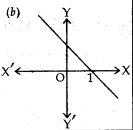Quadratic Polynomial (2 zeros) intersects X-axis at x = l.Quadratic Polynomial (no zero) Does not meet X-axis.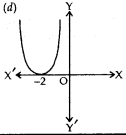Linear Polynomial (One zero) Passes through origin.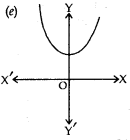Quadratic Polynomial (One zero) Meets X- axis at 2 points x = 1 and x = -lQuadratic Polynomial (2 zeros) Meets X- axis at 2 points x = 1 and x = -lLinear Polynomial (One zero) intersects X-axis at x = l.Linear Polynomial (One zero) Passes through origin.Quadratic Polynomial (one zero) Meets X- axis at -2Quadratic Polynomial (no zero) Does not meet X-axis.

Question 2.

 (a) p(x) = ax + b No. of Zeroes = 3 3 Zeroes αsv= – $$\frac{d}{a}$$ (b) q(x) = ax2 + bx +c (a ≠ 0) Cubic Polynomial 2 Zeroes Sum of the zeroes = 0 (c) r(x) = ax3 + bx2 + cx + d(a≠ 0) Linear Polynomial Meets X-axis at 3 α + s = –$$\frac{b}{a}$$Quadratic Polynomial One zero –$$\frac{b}{a}$$
 (a) p(x) = ax + b Linear Polynomial One zero –$$\frac{b}{a}$$ (b) q(x) = ax2 + bx +c (a ≠ 0) Quadratic Polynomial 2 Zeroes α + s = –$$\frac{b}{a}$$ (c) r(x) = ax3 + bx2 + cx + d(a≠ 0) Cubic Polynomial 3 Zeroes αsv= – $$\frac{d}{a}$$No. of Zeroes = 3 Meets X-axis at 3 Sum of the zeroes = 0